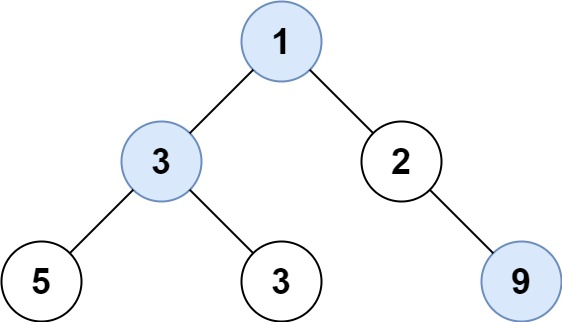# GeetCode Hub

Given the `root` of a binary tree, return an array of the largest value in each row of the tree (0-indexed).

Example 1:```Input: root = [1,3,2,5,3,null,9]
Output: [1,3,9]
```

Example 2:

```Input: root = [1,2,3]
Output: [1,3]
```

Example 3:

```Input: root = 
Output: 
```

Example 4:

```Input: root = [1,null,2]
Output: [1,2]
```

Example 5:

```Input: root = []
Output: []
```

Constraints:

• The number of nodes in the tree will be in the range `[0, 104]`.
• `-231 <= Node.val <= 231 - 1`

/** * Definition for a binary tree node. * public class TreeNode { * int val; * TreeNode left; * TreeNode right; * TreeNode() {} * TreeNode(int val) { this.val = val; } * TreeNode(int val, TreeNode left, TreeNode right) { * this.val = val; * this.left = left; * this.right = right; * } * } */ class Solution { public List<Integer> largestValues(TreeNode root) { } }